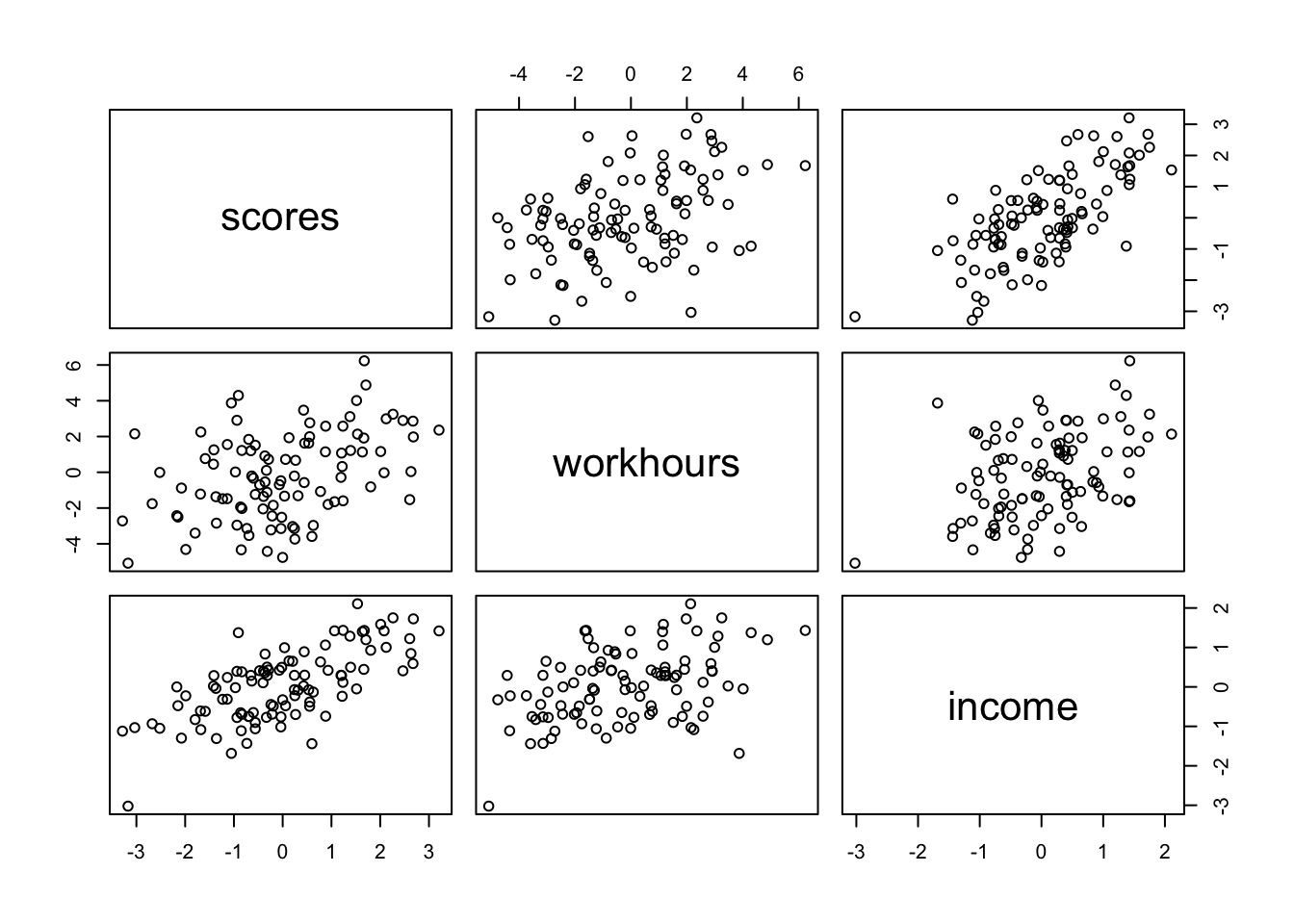## Chapter 5 - Many Variables and Spurious Waffles

This chapter introduced multiple regression, a way of constructing descriptive models for how the mean of a measurement is associated with more than one predictor variable. The defining question of multiple regression is: What is the value of knowing each predictor, once we already know the other predictors? The answer to this question does not by itself provide any causal information. Causal inference requires additional assumptions. Simple directed acyclic graph (DAG) models of causation are one way to represent those assumptions.

Place each answer inside the code chunk (grey box). The code chunks should contain a text response or a code that completes/answers the question or activity requested. Make sure to include plots if the question requests them. Problems are labeled Easy (E), Medium (M), and Hard(H).

Finally, upon completion, name your final output .html file as: YourName_ANLY505-Year-Semester.html and publish the assignment to your R Pubs account and submit the link to Canvas. Each question is worth 5 points.

## Questions

5E1. Which of the linear models below are multiple linear regressions? \begin{align} {μ_i = α + βx_i} \tag{1}\\ μ_i = β_xx_i + β_zz_i \tag{2} \\ μ_i = α + β(x_i − z_i) \tag{3} \\ μ_i = α + β_xx_i + β_zz_i \tag{4} \\ \end{align}

# 2, 3 and 4

5E2. Write down a multiple regression to evaluate the claim: Animal diversity is linearly related to latitude, but only after controlling for plant diversity. You just need to write down the model definition.

#μi = α + βAAi + βPPi
#where A is animal diversity and P is plant diversity

5E3. Write down a multiple regression to evaluate the claim: Neither amount of funding nor size of laboratory is by itself a good predictor of time to PhD degree; but together these variables are both positively associated with time to degree. Write down the model definition and indicate which side of zero each slope parameter should be on.

#μi = α + βFFi + βSSi
#βf and βs are positive.

5E4. Suppose you have a single categorical predictor with 4 levels (unique values), labeled A, B, C and D. Let Ai be an indicator variable that is 1 where case i is in category A. Also suppose Bi, Ci, and Di for the other categories. Now which of the following linear models are inferentially equivalent ways to include the categorical variable in a regression? Models are inferentially equivalent when it’s possible to compute one posterior distribution from the posterior distribution of another model. \begin{align} μ_i = α + β_AA_i + β_BB_i + β_DD_i \tag{1} \\ μ_i = α + β_AA_i + β_BB_i + β_CC_i + β_DD_i \tag{2} \\ μ_i = α + β_BB_i + β_CC_i + β_DD_i \tag{3} \\ μ_i = α_AA_i + α_BB_i + α_CC_i + α_DD_i \tag{4} \\ μ_i = α_A(1 − B_i − C_i − D_i) + α_BB_i + α_CC_i + α_DD_i \tag{5} \\ \end{align}

#Models 1, 3, 4, and 5 are inferentially equivalent.

5M1. Invent your own example of a spurious correlation. An outcome variable should be correlated with both predictor variables. But when both predictors are entered in the same model, the correlation between the outcome and one of the predictors should mostly vanish (or at least be greatly reduced).

N <- 100
income <- rnorm(n = 100, mean = 0, sd = 1)
workhours <- rnorm(n = N, mean = income, sd = 2)
scores <- rnorm(n = N, mean = income, sd = 1)
d <- data.frame(scores, workhours, income)
pairs(d)m <- map(
alist(
scores ~ dnorm(mu, sigma),
mu <- a + bo * workhours,
a ~ dnorm(0, 5),
bo ~ dnorm(0, 5),
sigma ~ dunif(0, 5)
),
data = d
)
precis(m)
##             mean         sd       5.5%     94.5%
## a     0.03446797 0.12819227 -0.1704080 0.2393440
## bo    0.23841153 0.05345272  0.1529838 0.3238393
## sigma 1.27893653 0.09043050  1.1344111 1.4234619
m <- map(
alist(
scores ~ dnorm(mu, sigma),
mu <- a + bo * workhours + bi * income,
a ~ dnorm(0, 5),
bo ~ dnorm(0, 5),
bi ~ dnorm(0, 5),
sigma ~ dunif(0, 5)
),
data = d
)
precis(m)
##              mean         sd        5.5%     94.5%
## a     -0.02824237 0.10011589 -0.18824690 0.1317622
## bo     0.08956287 0.04552220  0.01680961 0.1623161
## bi     0.97850827 0.12139611  0.78449384 1.1725227
## sigma  0.99565589 0.07040316  0.88313805 1.1081737

5M2. Invent your own example of a masked relationship. An outcome variable should be correlated with both predictor variables, but in opposite directions. And the two predictor variables should be correlated with one another.

N <- 100
rho <- 0.6
pressure <- rnorm(n = N, mean = 0, sd = 1)
pain <- rnorm(n = N, mean = rho * pressure, sd = sqrt(1 - rho^2))
sensitivity <- rnorm(n = N, mean = pressure - pain, sd = 1)
d <- data.frame(sensitivity, pressure, pain)
pairs(d)